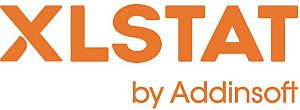# XLSTAT - Descriptive Statistics

A description of the sampling as well as an estimation of some statistics for the population is always useful to understand the distribution of the samples.

## Available descriptive statistics in XLSTAT

XLSTAT provides the following statistics:

 Quantitative data Qualitative data No. of values used No. of values ignored No. of min./max. value % of min./max. value Minimum/Maximum 1st quartile Median 3rd quartile Range: difference between the maximum and the minimum, Sum of the weights (if any) Total Mean Geometric mean Harmonic mean Kurtosis (Pearson), Skewness (Pearson) Kurtosis, Skewness CV (standard deviation/mean) Sample variance Estimated variance Standard deviation of a sample Estimated standard deviation Mean absolute deviation Standard deviation of the mean Summary for the variables: No. of categories Mode Mode frequency Mode weight % mode Relative frequency of the mode Statistics table for each category: Frequency Weight of the category %: percentage of the category Relative frequency of the category

Also some plots are very helpful to visualize the distribution.

 Charts created for quantitative variables Charts created for categorical variables Box plots, Scattergrams, Strip plots, Q-Q plots, p-p plots, Stem and leaf plots. Bar charts Pie charts Double pie charts Doughnuts Stacked bars Multiple barsThis analysis is available in the XLStat-Basic addin for Microsoft ExcelKovach Computing Services (KCS) was founded in 1993 by Dr. Warren Kovach. The company specializes in the development and marketing of inexpensive and easy-to-use statistical software for scientists, as well as in data analysis consulting.

### Get in Touch

• Email:
sales@kovcomp.com